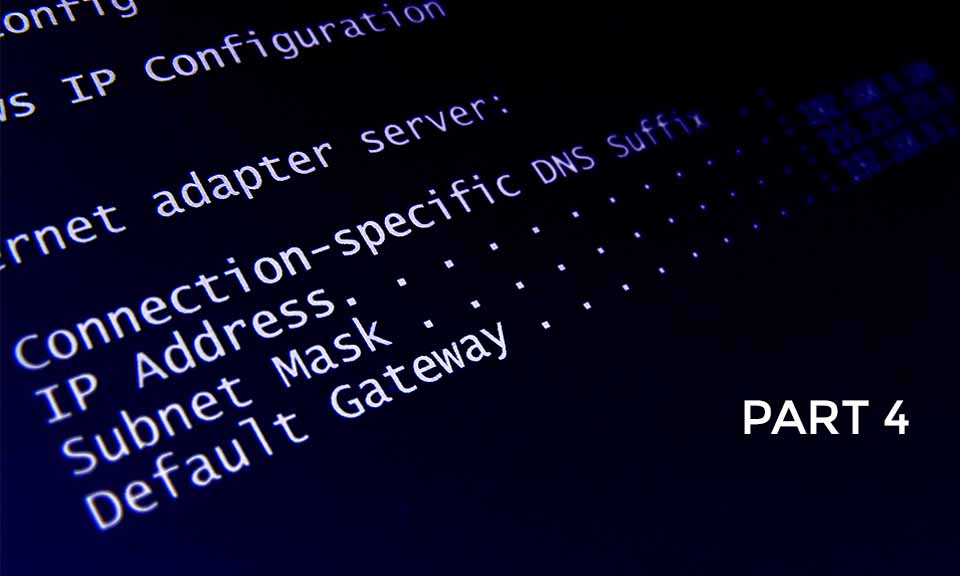## Blog Post# 4 – Classes of IPv4 Address

In IPv4 address, we have 5 classes namely Class A, Class B, Class C, Class D and Class E

but only 3 are usable i.e. Class A, Class B and Class C

## Class A

This class of IP Address is used for a network with large number of computers, most used by Large scale business

It has a subnet mask of 255.0.0.0, which means that the first octet represent the Network ID whiles the 2nd, 3rd and 4th octet represents the Host ID, so therefore is divided into two.

 255 .0.0.0 NID HID

Class B

This class of IP Address is used for a network with medium number of computers, most used by medium scale business

It has a subnet mask of 255.255.0.0, which means that the 1st and 2nd octet represent the Network ID whiles the 3rd and 4th octet represents the Host ID, so therefore is divided into two.

 255.255 .0.0 NID HID

## Class C

This class of IP Address is used for a network with small number of computers, most used by small scale business

It has a subnet mask of 255.255.255.0, which means that the 1st, 2nd and 3rd octet represent the Network ID whiles the 4th octet represents the Host ID, so therefore is divided into two.

 255.255.255 .0 NID HID

Class D

This class is mostly used for Multicasting by computer services or protocols

Class E

This class is mostly used for research and experiment

How do we detect the class of an IP Address automatically when we see it

Note this

### 0     0   0   0   0  0  0  0

This is an example of an IP Address (192.168.0 1). Our range

column below is based on every IPv4 address’ 1st octet

 Class Binary Bit Range Understanding Class A 10000000 1 – 126 Note: the 1st binary bit is turned ON and that represents 128 (We can’t start at 0 so we start at 1 and also we can’t end at 128 because it starts the next range, also we can’t end at 127 because it represents our loopback address of 127.0.0.1, so we end at 126 Class B 11000000 128 – 191 Note: We already start at 128 because class A ends at 126. Here, the 1st and 2nd binary bit are turned ON and that adds up to 192 (We can’t end at 192 because it starts the next range, so we end at 191 Class C 11100000 192 – 223 Note: We already start at 192 because class B ends at 191. Here, the 1st. 2nd and 3rd binary bit are turned ON and that adds up to 224 (We can’t end at 224 because it starts the next range, so we end at 223 Class D 11110000 224 – 239 Note: We already start at 224 because class C ends at 223. Here, the 1st. 2nd, 3rd and 4th binary bit are turned ON and that adds up to  240 (We can’t end at 240 because it starts the next range, so we end at 239 Class E 11111000 240 – 248 Note: We already start at 240 because class C ends at 239. Here, the 1st. 2nd, 3rd, 4th and 5th binary bit are turned ON and that adds up to 248 (We end at 248 because no more classes)

Example 1

Now when you see a random IP Address like 34.21.2.1 you should know it is a class A because for Class A address, their 1st octet falls between 1 to 126

So automatically it’s subnet mask becomes 255.0.0.0 because it is a class A address.

Class A

### 255.0.0.0

Example 2

Now when you see a random IP Address like 140.21.2.1 you should know it is a class B because for Class B address, their 1st octet falls between 128 to 191

So automatically it’s subnet mask becomes 255.255.0.0 because it is a class B address.

Class B

### 255.0.0.0

Example 3

Now when you see a random IP Address like 195.21.2.1  you should know it is a class C because for Class C address, their 1st octet falls between 192 to 223

So automatically it’s subnet mask becomes 255.255.255.0 because it is a class C address.